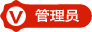#### WIN10系统下被坑的FreeBasic代码 VFB2019-5-25 2523

```  Open 文件名 For Binary Access Write As #M
If Put(#M, 地址, by()) = 0 Then Function = True Else Function = False```

```  Open 文件名 For Binary As #M
If Put(#M, 地址, by()) = 0 Then Function = True Else Function = False```